• 要说明是，现在这篇东西是研究用椭圆曲线精确判定一个正整数是否为素数，而不是研究用椭圆曲线加解密！当然，后面提供一些关于有限域上椭圆曲线上加法，乘法，除法实装函数都是可以直接用于加解密...
要说明的是，现在这篇东西是研究用椭圆曲线精确判定一个正整数是否为素数，而不是研究用椭圆曲线加解密的！
当然，后面提供的一些关于有限域上的椭圆曲线上的点的加法，乘法，除法的实装函数都是可以直接用于加解密的。
这次要研究的ECPP，它是接我以前的一篇关于素数的blog（http://blog.csdn.net/chen09/archive/2008/03/24/2214219.aspx
），“试除法”以外的素数精确判定的方法之一，它的算法复杂度为：O((log n)^6)。

“有限域上的椭圆曲线”，这个概念的子概念，顾名思义有两个：1，有限域，2，椭圆曲线。这两个子概念都很容易理解，但是合在一起，定义在有限域上的椭圆曲线，而不是定义在普通的实数上的椭圆曲线，是怎么一回事儿？我大概搞了整整一个月才明白。网上的中文和日文资料都太少了，而且错误百出，让我走了不少弯路。在这里想把我所知道的写清楚，让后来人可以多一份可以参考的资料。

什么是椭圆曲线？什么是有限域？什么是定义在有限域上的椭圆曲线？它有什么特征？和判断素数有什么关系？
这里有一篇《ECC加密算法入门介绍》（http://www.docin.com/p-853208.html
），写得非常的好，错误几乎没有。通读一边有益无损。
初中时，我们就学过，方程x^2+y^2=1是个圆，以(0,0)为中心，半径为1。
稍作变形，方程(x-a)^2+(y-b)^2=c^2也是个圆，以(a,b)为中心，半径为c。
然后说说椭圆方程，(x/a)^2+(y/b)^2(a>b>0)是个标准方程。
稍作变形，((x-h)/a)^2+((y-k)/b)^2=1。h,k自然是x轴和y轴上的位移了。
有没有都回想起来呢？如果回想起来了，那么把它们都忘了吧！因为椭圆曲线和椭圆方程没有什么关系。8-)

《ECC加密算法入门介绍》（http://www.docin.com/p-853208.html
）里面讲椭圆曲线时先介绍了射影平面，这个有点超纲（在我看来，所有计算机的题目都是用初中数学可以解决的，凡是用到高中数学以上的东西，都是超纲的）。所以还是直接考察笛卡尔坐标系上的，椭圆曲线：y^2+a1*x*y+a3*y=x^3+a2*x^2+a4*x+a6。
看上去有点复杂？其实已经很简单化了！要知道：两个元（x和y）且是3次的一般方程要复杂的多。而现在要求每个点都是光滑的才是椭圆曲线，所以方程的形式真的已经简单很多。
还是觉得有点复杂？没关系，实际运用中的椭圆曲线的形式将更为简单。

现在，这个椭圆曲线是定义在实数上的，对于曲线上的点都可以求导（因为我们要求它是光滑的），求导的方法很简单，两边分别对y和x求偏导就可以。求导自然是为了就切线，为什么要算切线？稍微过一会儿说。

现在说一下，曲线上的2个点的加法。
什么是加法？
大家都知道1+1=2(不是哥德巴赫猜想，是小学或者说幼儿园的数学)，但是1+1为什么等于2？因为这个“+”是人为定义的一个算法，在首先自然数上定义，然后扩展到整数，有理数，实数，复数。

罗素（Bertrand Russell, 1872-1970）和他的导师怀特黑(Alfred Whitehead, 1861-1947)用了几十页的篇幅，最终成功地证明1+1=2（参见，若奇的《孤独的破译者和他的计算机器》 http://xys4.dxiong.com/xys/netters/psi1b/ruoqi.txt
）。

现在，天才的数学家们也定义了椭圆曲线上的两个点的加法！
令椭圆曲线两个点P，Q，做一条过点P和点Q的直线（如果P，Q重合，则做P点上的切线（这就是刚才说到为什么要求导求切线的原因）），交椭圆曲线上的另一点R'，过R'做y轴的平行线交椭圆曲线与点R。规定：P+Q=R!

为什么要定义这么个不着边际的运算法则？
的确，它离我们的目标，无论是精确素数判断还是费马大定理都离得很远。
这里，我想可以运用人择原理：如果它是没有用的，那么就不会被我们注目；虽然它不是那么直观，但是它是有用的，所以我们现在在学习它。

就好象，1+1=2，原来也许没有什么用，但是要计算1个苹果加上1个生梨，共有几个水果时，起到了作用，所以1+1=2被保留了下来。
1+1威力如此之大，不但用于幼儿的数学启蒙，也创造了诺贝尔奖获得者罗素！大家都知道诺贝尔是没有数学奖的，罗素得到的是文学奖。罗素和怀特黑的两千多页的《数学原理》（和牛顿的伟大的著作同名，说明他们有如此的自信）耗尽了他的心血，哥德尔(Kurt G?del, 1906-1978)和他的“哥德尔不完备定理”的出现，使罗素则彻底丧失了对数理逻辑的兴趣，他改而倾心哲学和文学，关注社会事务。终于，在1950年他获得了诺贝尔文学奖。

1+1威力还不止于此，同样因为哥德尔的出现，也使冯·诺依曼(John von Neumann, 1903-1957)放弃了纯粹数学的研究。1944年，他与奥斯卡·摩根斯特恩合著出版《博弈论与经济行为》，标志着现代系统博弈理论的的初步形成，从而被称为“博弈论之父”；1945年6月，他戈德斯坦、勃克斯等人联名发表了著名的“101页报告”，而成了现代计算机之父。

哥德尔是有颠覆性的，他的不完备定理最初可以描述为“如果将算术运算公理引入一阶谓词系统，则由此而成的算术运算系统是不完备的，即存在这样的命题，它们的真伪是不可证明的。”而一阶谓词显然与50年后（20世纪70，80年代）的计算机届有直接的关系，以日本为首的推崇的第5代计算机就是以Prolog为主要语言，而Prolog就是以一阶谓词逻辑为基础的。虽然第5代计算机以失败而告终，但是不得不佩服日本敢为天下先的勇气，如果成功，那么我们现在的世界也许完全不是现在这个样子的了。

扯了半天，想说明的就是，P+Q=R!

定义在实数上的椭圆加法，还有图像可以看，但是定义在有限域上的椭圆曲线及其加法，就很难理解了。杨振宁曾说过，现在的数学论文，有的是看了第一页就看不下去的；有的是看了第一句就看不下去的。幸亏，有限域上的椭圆曲线还是可以看下去的，虽然它比“1个苹果加上1个生梨”要复杂些。但是只要有了抽象的头脑，它并不比“2头牛加上3头羊等于5头动物”要难多少。

刚才，我们把椭圆曲线定义在实数上，它是连续的。现在我们把它定义在有限域上。
有限域的定义很简单：包含有限个元素的域被称为有限域。

如果从有限域扯开去，那么和我们熟知的一些数学和计算机都有很大关系。比方说，每个方程对应于一个域，即含有方程全部根的域，称为这方程的伽罗瓦域，这个域对应一个群，即这个方程根的置换群，称为这方程的伽罗瓦群。伽罗瓦域的子域和伽罗瓦群的子群有一一对应关系；当且仅当一个方程的伽罗瓦群是可解群时，这方程是根式可解的。
这个理论是不是很难理解？没有关系，它的推论就容易理解的多：1，可以得出五次以上一般代数方程沒有公式解（华罗庚曾经指出过某人的5次方程公式解的错误）；2，尺规作图三等分任意角和作倍立方体不可能；等等。

不过我们不需要知道那么多。我们只要知道定义在有限域上的椭圆曲线就是：
那些点P或者Q的坐标(x,y)都不是实数范围那么大，而是有限的。比方说，（0，1，2，3，4）或者（0，1，2，3，4，5，6）。注意到，这连个范围的个数5或者7都是素数！

刚才说到，椭圆曲线函数太复杂了，实际运用中，我们用比较简单的形式：y^2 = x^3 + a*x +b 。（含有x*y项的椭圆曲线方程在这篇文章里面被俺完全忽略了，有兴趣的可以参阅网上的文章或者自己推导一下，公式比不含x*y项的要复杂一些，但是原理是一样的）
然后，很容易写出个程序，把这个方程的解都算出来。

但是，说到这里要稍微等等。我们虽然把椭圆函数定义到了有限域上，但是这时候有限域的威力还没有发挥。我们把有限域的个数（素数）也放到椭圆曲线方程里面去！
y^2 = x^3 + a*x +b (mod p)，p为素数，x,y 属于 (0，1，...，p-1)
好了！现在我们把有限域，椭圆曲线合成起来了。
举个例子，我们来最简单的y^2 = x^3 + x +1 (mod 5)
下面就是所有的解：
[x=3 y=1]
[x=3 y=4]
[x=4 y=3]
[x=4 y=2]
[x=2 y=1]
[x=2 y=4]
[x=0 y=4]
[x=0 y=1]
要验算很简单吧？把上面的x和y代入上面的方程，看看左右是否相等。别忘记，方程左右要对5取余。

那么上面的解，也就是椭圆曲线的各个点，P+Q=R这个加法怎么办呢？形式上很像的：
加法： a+b ≡ c (mod p)
乘法： a*b ≡ c (mod p)
除法： a/b ≡ c (mod p) 即 a * b^(-1) ≡ c (mod p)

乘法？第一次听说吧？椭圆曲线上的点和点是没法相乘的，这里的乘法就是连加，和初等数学类似。a*b 也就是 a + a + ... + a ，一共b个a相加。
乘法理解后，除法也就容易了，a/b也就是 a * b^(-1)，而b^(-1)也就是要求出一个数x（0<= x <p）让x*b ≡ 1 (mod p)
同样是y^2 = x^3 + x +1 (mod 5)
加法的例子：
[x=3 y=1] + [x=4 y=3] = [x=2 y=1]

乘法的例子：
[x=3 y=1] * 2 = [x=3 y=1] + [x=3 y=1] = [x=0 y=1]
[x=3 y=1] * 3 = [x=3 y=1] * 2 + [x=3 y=1] = [x=0 y=1] + [x=3 y=1] = [x=2 y=4]

这里的加法就是前面定义过的加法，那个画通过被加的2个点的直线，然后相交，然后再画平行y轴的直线，再相交的那个点，就是加法的结果。算法虽然比较复杂，一般公式推导却可以自己来。这里就不推导了，直接给出一般公式：
椭圆曲线： y^2 = x^3 + a*x +b (mod p)
P(x1,y1) + Q(x2,y2) = R(x3,y3)
x3 ≡ λ^2 - x1 - x2  (mod p)
y3 ≡ λ * (x1 - x3) - y1 (mod p)
其中
如果，PQ重合，则 λ ≡ (3 * x^2 + a) / (2 * y) (mod p)
PQ相异，则 λ ≡ (y2-y1)/(x2-x1) (mod p)
要注意，上面的除法，是我们刚刚定义的除法（就是除数乘以结果的对p取余等于被除数对p取余），而不是实数上的除法。
有了乘法，我们再引入一个概念，阶（或者秩（不是线性代数行列式里面的秩）或者级数）。
我们还是拿最简单的 y^2 = x^3 + x +1 (mod 5) 来做例子。
最简单的一个解P[x=0 y=1]，我们来计算，P * 2，P * 3，...
P * 1 : [x=0 y=1]
P * 2 : [x=4 y=2]
P * 3 : [x=2 y=1]
P * 4 : [x=3 y=4]
P * 5 : [x=3 y=1]
P * 6 : [x=2 y=4]
P * 7 : [x=4 y=3]
P * 8 : [x=0 y=4]
那么P * 9是多少？也许有人说，岂不是很简单？套用上面的公式就可以。但是......
P * 9 = P * 1 + P * 8，P * 1 和 P * 8的x都是0，也就是说通过它们的直线是垂直于x轴，平行于y轴的，就不能和椭圆曲线再相交了！用公式套用时，λ算不出了。
同样，P * 9 = P * 2 + P * 7 = P * 3 + P * 6 = ......可惜，P * 2 和 P * 7的x是一样的，P * 3 和 P * 6的x也是一样的。也就是说P * 9是不存在的，或者说交点在无限远。

而，这个数字9就是就是点P[x=0 y=1]的阶。

重新定义一下，椭圆曲线上的某个点的阶n就是，使P*n等于无限远。
上面关于 y^2 = x^3 + x +1 (mod 5)的点[x=0 y=1]的阶，可以参考下面的链接《椭圆曲线密码系统》的第11页。http://www.docin.com/p-17972799.html
但是很可惜，这个链接里面P * 3算错了，应该是[x=2 y=1]，而不是[x=4 y=2]。

大家是不是有点头晕了？至少我自学到这里，已经非常头晕了。但是，再忍一忍，我们的素数判断算法呼之欲出了。那就是：
定义在有限域p上的椭圆曲线上的每个点（解），必然有阶！
具体的证明就不写了，我们也不感兴趣，我们只要结论，只要有可操作的方法。
现在，we got it。

从头开始整理一遍：
1，简单的椭圆曲线：y^2 = x^3 + a*x +b (mod p)
2，我们可以取a = 1, b = 1 ，但是必须要保证 4*a^3 + 27*b^2 mod p 不等于零。比方说，我们要判定31是不是素数时，就不能用y^2 = x^3 + x + 1 (mod 31)，这个时候，我们可以取a = 1, b = 2。
3，我们计算出所有的解P(x,y)，其中 0 <= x < p，0 <= y < p。
4，计算每个解P(x,y)的乘法P * n , 其中n从2开始往上递增。每次计算出来的P * n的x值都保存起来，如果后来计算出来的P * n的x值和前面重复了，那就说明找到了这个解的阶，这个时候处理下一个解，如果所有的解都处理了，都找到了阶，那就这个p就是素数；如果计算P * n是发生了错误，一般来说，就是计算λ时，那个除法没有解，那么这个p就是合数（不是素数）。

上面就是计算的流程了。

然后，就让我们把它们变成Java的代码吧。

首先，先定义一下简单的Exception，当计算出错时可以被抛出。

package elliptic;
public class EllipticException extends Exception {
/**
*
*/
private static final long serialVersionUID = 3528609824516918284L;
public EllipticException() {
}
public EllipticException(String message) {
super(message);
}
public EllipticException(Throwable cause) {
super(cause);
}
public EllipticException(String message, Throwable cause) {
super(message, cause);
}
}

这个没有什么好解释的吧？

然后我们来定义椭圆曲线：

package elliptic;
/**
*
* y^2 = x^3 + a*x +b (mod p)
* @author z1j2110
*
*
*/
public class Line {
int a;
int b;
int p;
static public Line newInstance(int a, int b, int p) throws EllipticException {
return new Line(a, b, p);
}
private Line(int a, int b, int p) throws EllipticException {
if ((4 * a * a * a + 27 * b * b) % p == 0)
throw new EllipticException("4*a^3 + 27*b^2 == 0 mod p : a=" + a + "b=" + b + "p=" + p);
this.a = a;
this.b = b;
this.p = p;
}

@Override
public String toString() {
return "[a=" + a + " b=" + b + " p=" + b + "]";
}
}

这个Line类就是我们的椭圆曲线了，里面的a,b,p都解释过了，还有印象吧？

然后就是解（点）了：
package elliptic;
public class Point {
int x;
int y;
static Point newInstance(int x, int y) {
return new Point(x, y);
}
Point(int x, int y) {
this.x = x;
this.y = y;
}
@Override
public boolean equals(Object obj) {
if (!(obj instanceof Point))
return false;
Point other = (Point) obj;
if (this.x != other.x)
return false;
if (this.y != other.y)
return false;
return true;
}
@Override
public String toString() {
return "[x=" + x + " y=" + y+"]";
}

}

这个也没有什么好说的，就是点(x,y)而已。

关键的东西来了：

package elliptic;
import java.util.Collections;
import java.util.HashMap;
import java.util.HashSet;
import java.util.Map;
import java.util.Set;
public class Elliptic {
static private Map<Integer, Point> mapResult = Collections.synchronizedMap(new HashMap<Integer, Point>());
static public boolean isPrime(int x) {
Line l = null;
int i = 1;
while (l == null) {
try {
l = Line.newInstance(1, i, x);
} catch (EllipticException e) {
i++;
}
}
Set<Point> set = getSolution(l);
for (Point p : set) {
if (p.y == 0)
continue;
int n = 0;
Set<Integer> setX = Collections.synchronizedSet(new HashSet<Integer>());
try {
while (true) {
Point point;
point = multiply(p, ++n, l);
if (setX.contains(point.x)) {
break;
} else {
}
}
} catch (EllipticException e) {
return false;
}
}
return true;
}
static public Set<Point> getSolution(Line l) {
Set<Point> set = Collections.synchronizedSet(new HashSet<Point>());
for (int x = 0; x < l.p; x++) {
for (int y = 0; y < l.p; y++) {
Point p = Point.newInstance(x, y);
if (isSolution(p, l))
}
}
return set;
}
static public boolean isSolution(Point p, Line l) {
int left = (p.y * p.y) % l.p;
int right = (p.x * p.x * p.x + l.a * p.x + l.b) % l.p;
if (right < 0)
right += l.p;
return left == right;
}
static public Point plus(Point p, Point q, Line l) throws EllipticException {
// if (!isSolution(p, l))
// throw new EllipticException(p + " is not the solution of line " + l);
//
// if (!isSolution(q, l))
// throw new EllipticException(q + " is not the solution of line " + l);
int k = lambda(p, q, l);
int x3 = (k * k - p.x - q.x) % l.p;
if (x3 < 0)
x3 += l.p;
int y3 = (k * (p.x - x3) - p.y) % l.p;
if (y3 < 0)
y3 += l.p;
return new Point(x3, y3);
}
static public Point multiply(Point p, int x, Line l) throws EllipticException {
mapResult.clear();
// mapResult.put(1, p);
return multiply(p, x, l, mapResult);
}
static private Point multiply(Point p, int x, Line l, Map<Integer, Point> mapResult)
throws EllipticException {
if (x == 1)
return p;
if (mapResult.containsKey(x))
return mapResult.get(x);
int half = x / 2;
while (half > 0) {
try {
Point point = plus(multiply(p, half, l, mapResult), multiply(p, x - half, l,
mapResult), l);
mapResult.put(x, point);
return point;
} catch (EllipticException ee) {
half--;
}
}
throw new EllipticException("can't multiply " + p + "*" + x + " mod " + l.p);
}
static public int lambda(Point p, Point q, Line l) throws EllipticException {
if (p.x == q.x) {
if (p.y == q.y) {
return (3 * p.x * p.x + l.a) * inverse(2 * p.y, l.p);
} else
throw new EllipticException("p+q=∞ p=(" + p + ")q=(" + q + ")");
} else
return (q.y - p.y) * inverse(q.x - p.x, l.p);
}
static public int inverse(int x, int p) throws EllipticException {
while (x < 0)
x += p;
for (int i = 0; i < p; i++) {
if (i * x % p == 1)
return i;
}
throw new EllipticException(x + " * x == 1 mod " + p + " x is not exist.");
}
}

这个就是我们的主要的功能类了。稍微解释一下。

static public boolean isSolution(Point p, Line l)
这是用来判断，点p是否是椭圆曲线l的解？

static public Set<Point> getSolution(Line l)
这是用来计算椭圆曲线的所有解得函数，返回值是个集合，里面的元素是Point点

static public int lambda(Point p, Point q, Line l) throws EllipticException
顾名思义，这是计算λ值的函数。

static public int inverse(int x, int p) throws EllipticException
顾名思义，这是计算除法时要用到，求(^-1)的函数。

static public Point plus(Point p, Point q, Line l) throws EllipticException
顾名思义，这是加法。

static public Point multiply(Point p, int x, Line l) throws EllipticException {
mapResult.clear();
// mapResult.put(1, p);
return multiply(p, x, l, mapResult);
}
static private Point multiply(Point p, int x, Line l, Map<Integer, Point> mapResult)
throws EllipticException {
if (x == 1)
return p;
if (mapResult.containsKey(x))
return mapResult.get(x);
int half = x / 2;
while (half > 0) {
try {
Point point = plus(multiply(p, half, l, mapResult), multiply(p, x - half, l,
mapResult), l);
mapResult.put(x, point);
return point;
} catch (EllipticException ee) {
half--;
}
}
throw new EllipticException("can't multiply " + p + "*" + x + " mod " + l.p);
}

顾名思义，这是乘法。
稍微说明一下，这里用到了递归。因为显然当计算P*n时，n非常大的话，n个P连加也是很费时间的，所以要用点乘法，学过CPU内核设计的都知道。不记得的话，回去看看本科计算机的《硬件原理》。
比方说要计算P*9，就是计算P*4+P*5；计算P*4，就是计算P*2+P*2；P*2 = P+P；P*5 = P*2+P*3；P*3 = P*1+P*2；把计算出的中间结果保存在mapResult中，可以反复利用，以加快速度。

最后，我们的判定函数就是：
static public boolean isPrime(int x) {
Line l = null;
int i = 1;
while (l == null) {
try {
l = Line.newInstance(1, i, x);
} catch (EllipticException e) {
i++;
}
}
Set<Point> set = getSolution(l);
for (Point p : set) {
if (p.y == 0)
continue;
int n = 0;
Set<Integer> setX = Collections.synchronizedSet(new HashSet<Integer>());
try {
while (true) {
Point point;
point = multiply(p, ++n, l);
if (setX.contains(point.x)) {
break;
} else {
}
}
} catch (EllipticException e) {
return false;
}
}
return true;
}
如果你耐心的看到这里，上面的函数一点不难理解。
就是上面的4步的流程了。

然后让我们来写一段测试用的代码：
public static void main(String[] args) throws EllipticException {

for (int i = 10; i < 1000; i++) {
boolean isPrime = Elliptic.isPrime(i);
if (isPrime)
System.out.print(i + " ");
}
System.out.println();
}
}

这是用来，输出所有的10到1000之间的素数。结果为：
11 13 17 19 23 29 31 37 41 43 47 53 59 61...... 947 953 967 971 977 983 991 997
大家可以验证一下。

最后，说说算法复杂度。
首先要算出椭圆曲线的解，那就是n^2了，因为x从0到p-1，y也从0到p-1，逐一找出解。
然后，对于每个解都要看有没有阶，为了得到阶，要计算P*n，所以又多了个n^1，当然我们用了点乘法，所以是log2的n^1
然后就是，加法时要计算除法，除法时要从1到p检测，所以又有个n^1。
我的算法的复杂度是O((log n)*n^3)。说实话，我也不知道O((log n)^6)（应该是正解）这个值是怎么来的。
呵呵。

题外的一些话：
1，在相同的安全强度下，ECC的密钥长度和处理速度比RSA要短且快，随着RSA被破解，以后或许会是ECC的世界，它更适合放在IC卡或者手机里。在日本，上网的手机用户已经超过PC用户，以后网上商店或者网上拍卖的网站，将越来越倾向于移动用户，所以ECC应该大有可为。
2，费马大定理，也称费马最后定理（当整数 n>2 时，关于x,y,z的不定方程：x^n+y^n=z^n的自然数解是不存在的）的被证明过程中，诞生了代数几何上的椭圆曲线。在计算机上最大的应用就是前面讲的非对称加密。在数学上，谷山·志村猜想（椭圆曲线和模形式一一对应）的最后被证明直接可以反证出费马大定理。（因为可以证明，如果x^n+y^n=z^n n>2有自然数解，谷山·志村猜想将不成立）。
展开全文算法 exception string lambda class
• ## 圆桌理论

千次阅读 2020-01-17 23:32:54
圆桌理论 是网络游戏《World of Warcraft》(《魔兽世界》)中关于攻击判定的一个理论。来源于“一个圆桌面积是固定，如果几件物品已经占据了圆桌所有面积时，其它物品将无法再被摆上圆桌”。 2.攻击命中...
1.圆桌理论（what）
圆桌理论 是网络游戏《World of Warcraft》(《魔兽世界》)中关于攻击判定的一个理论。来源于“一个圆桌的面积是固定的，如果几件物品已经占据了圆桌的所有面积时，其它的物品将无法再被摆上圆桌”。
2.攻击的命中判定
为了方便、这里只考虑躲闪，招架，致命攻击、普通攻击四种结果、按优先级排序
躲闪–>招架–>致命攻击–>普通攻击
3.概率论算法
概率论算法是最常见的处理方式
思想：先判定是否躲闪，再判定是否招架，之后判断是否致命一击，最后才是普通攻击。
例如：
目标的躲闪几率……20%
目标的招架几率……5%
战士的致命一击率……30%
结果
1、目标躲闪此次攻击，几率为20%
2、目标招架此次攻击，几率为（1-20%）*5%，计算得到的值是4%，要低于目标的原始5%招架几率
3、目标致命一击，几率为(1-20%-（1-20%）*5%)*30%=22.8%，小于原始30%致命一击率
4.目标普通攻击，几率为(1-20%-（1-20%）5%)（1-30%）=53.2%
优点
无论如何都会得到可行的结果，最终各种情况所占的比例加起来可以涵盖到并且只能涵盖到一个样本范围，在物理上可行
缺点
逐级判定使得各属性有了额优劣之分，不能平等的影响最终出现的结果，优先级越高的属性增幅受益越高，优先级越低增幅所受的衰减越大，比如每提高躲闪1%，就能实实在在的提高1%的几率不被攻击，然而提高1%招架的时候，只能提高（1-躲闪几率）*1%的几率不被战士攻击到。
4.圆桌理论算法
圆桌理论算法就是为了将所有属性尽可能平等的考虑，保证每一个影响因素都能在统计中呈现出原始的出现几率，
思想：属性仍具有优先级，想象一个容量有限的桌子（100%），尽可能地让属性概率按优先级进入桌子，直至塞满整个桌子（100%）
例：还是同样的例子
目标的躲闪几率……20%
目标的招架几率……5%
战士的致命一击率……30%
三个概率进入桌子，占据55%，剩下的45%用普通攻击填补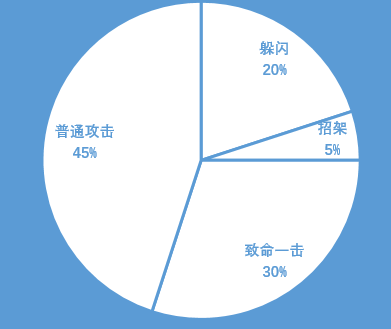如果躲闪概率上升到70%
目标的躲闪几率……70%
目标的招架几率……5%
战士的致命一击率……25%
那么致命一击只有25%能进入桌子，（被吃掉了5%），而普通攻击根本不会出现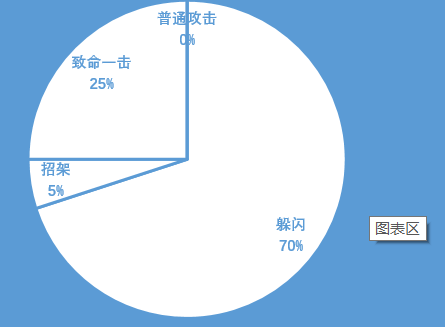可以看出，圆桌理论通过牺牲优先级低的属性的概率，来保证其他的属性能显现出原始的概率
优点
1.只需要判定一次，不需要逐级判定（减少了计算开销）
2.一定程度上保证属性互相平等
缺点
仍然引入了属性的优先级，牺牲了一部分属性的概率，在某些极端情况下，某些因素永远也无法发生，这是极其残忍且不符合道理的。（并且会发生闪避过高吞掉暴击概率的奇妙情景）


展开全文• 关于天线辐射电磁波极化类型判断原则是这样: 理想情况下, 天线辐射极化只有一种, 我们称之为主极化. 但实际情况下, 由于天线设计, 加工装配和工作环境等方面原因, 致使天线在辐射主极化分量同时, 还辐射...
• 1. 关于Harris角点检测采用特征值作为判定标准思考 窗口滑动像素灰度值变化函数E方程实际上是二次型（网上很多文章介绍该函数具体形式和由来，这里不再赘述，见参考资料）。主对角线元素（特征值）恒大于零...
1. 关于Harris角点检测采用特征值作为判定标准的思考
窗口滑动的像素灰度值变化函数E的方程实际上是二次型（网上很多文章介绍该函数的具体形式和由来，这里不再赘述，见参考资料1）。主对角线元素（特征值）恒大于零且不相同，那么该二次型代表的是椭圆。二次型中间矩阵特征值分解后，对角矩阵代表对圆的伸缩，两边正交矩阵代表对椭圆的旋转（参考马同学对特征值分解的介绍）。两个特征值分别对应椭圆的长轴、短轴。两个特征值分别是椭圆长、短轴平方的倒数。即轴长度与特征值成反比例关系（计算一下标准椭圆的二次型矩阵就明白了）。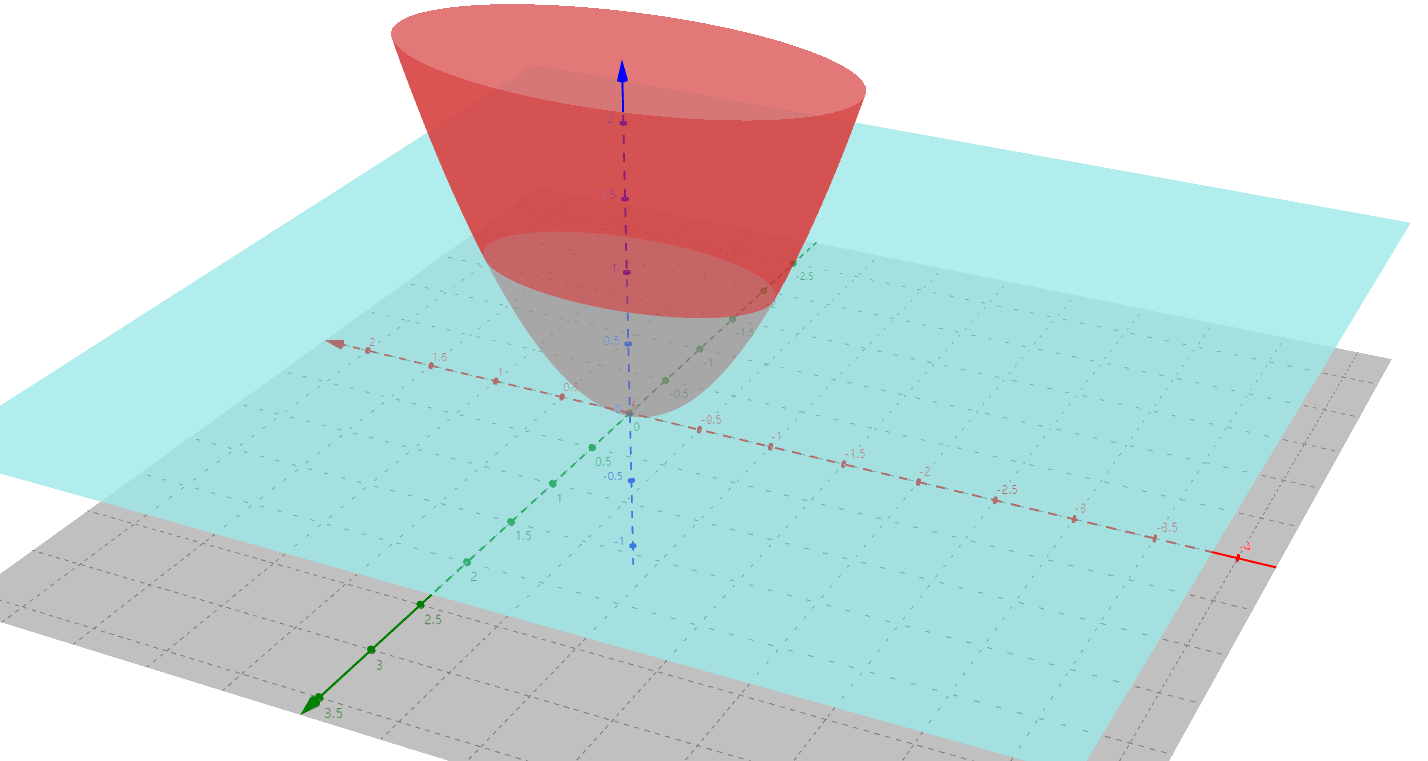图中红色图像是灰度值变化函数 E 的图像，其形状类似倒立的圆锥体，但是每一个水平截面都是椭圆曲线（非实心椭圆区域），且长、短轴正比于sqrt(z)。灰色平面是 X-Y 平面。蓝色平面是 Z=c（常数）即 E=c 平面。

假定给定灰度值变化量，即令函数E=c（常数）。该二次型函数变成一个椭圆方程。如下图：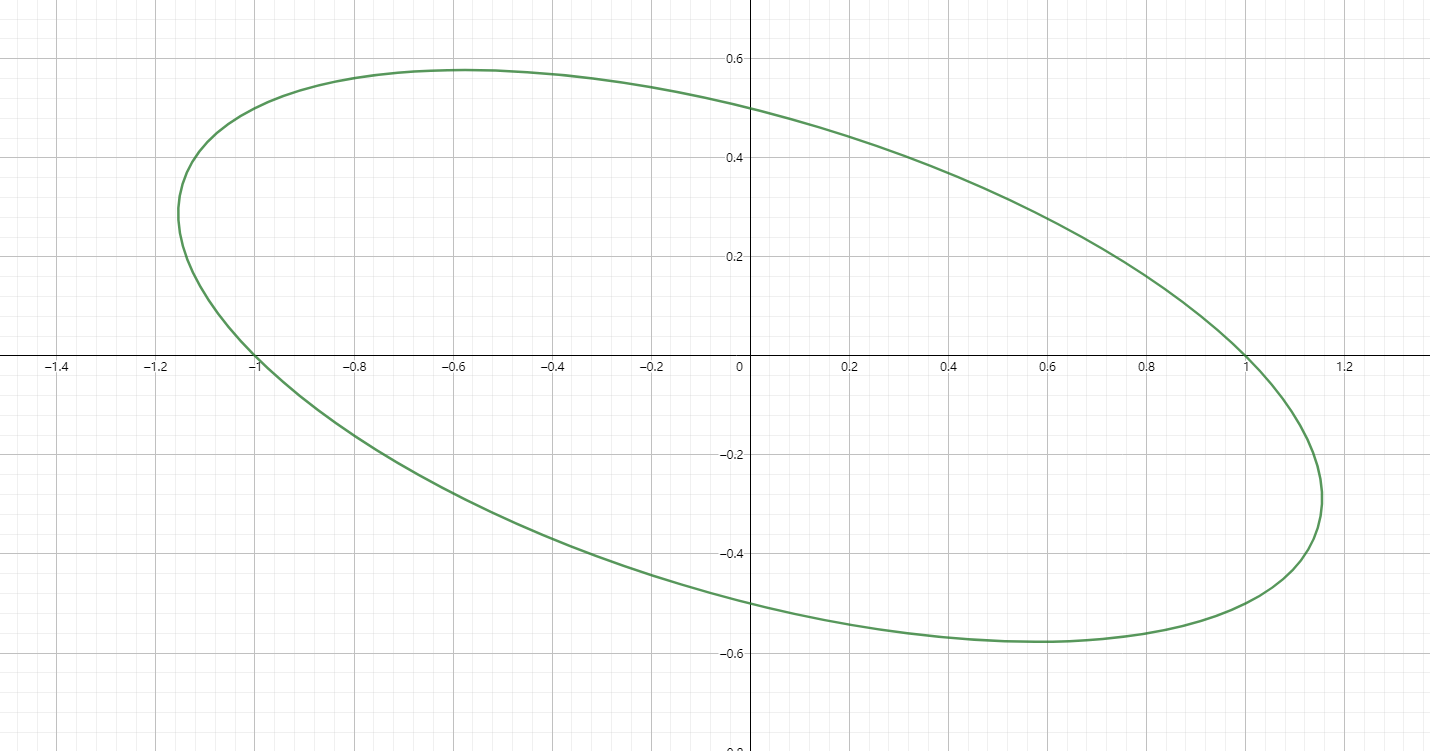此时，该二次型代表以偏移量（u，v）为变量的椭圆区域。椭圆短轴的地方，代表像素值变化最剧烈的方向，因为得到相同灰度变化值所需的偏移量最小；长轴的地方，代表像素值变化最缓慢的地方，因为得到相同灰度变化值所需的偏移量最大。
椭圆长短轴分三种情况：
（1）如果长短轴都很大且相近，代表着所有方向的变化都很缓慢，证明窗口在平坦区域；
（2）如果长轴很长，断轴很短，代表在单一方向像素值变化快，其他方向像素值变化慢，证明窗口内包含边缘；
（3）如果长、断轴都很小且相近，代表两个（多个）方向的像素值变化都很快，证明窗口内包含角点。

2. 为什么Harris矩阵M代表的二次型一定是椭圆呢？
我们知道二次型与圆锥曲线密切相关。
M矩阵进行特征分解后的两个特征值为
$\lambda_{1} , \lambda_{2}$
对角矩阵
$\begin{bmatrix} \lambda_{1} &0 \\ 0 & \lambda_{2} \end{bmatrix}$
表征M代表的图形的具体形状，而单位正交特征向量矩阵则代表对该形状的旋转。两个特征值分别是椭圆长、短轴平方的倒数。
要证明M矩阵代表的必定是椭圆，需要证明如下两条：
1、M矩阵有两个不同的特征值，那么M才有两个正交的特征向量。
2、M矩阵的两个特征值均大于0。（这条和椭圆方程形式有关，若一正一负表示双曲线。均为负，该二元二次函数代表的图像与平行于X-Y平面的截面也是椭圆，只不过图像在X-Y平面下方，其函数值为负，与Harris中用二次型代表窗口滑动后像素点灰度值变化程度相悖，因为该程度取欧氏距离（L2范数）的平方，恒为正。）
M矩阵为
$\begin{bmatrix} A &B \\ B & C \end{bmatrix}$
其中A、B、C分别为：
$A=\sum_{m}I_{x_{m}}^{2} \ \ ,C=\sum_{k}I_{y_{k}}^{2}\ \ ,B=\sum_{i}I_{x_{i}} I_{y_{i}}$
其中，Ixi表示窗口在（xi,yi）点处像素值对x轴的偏导数。
由A、B、C的定义可知，A>0，C>0。

3. 证明：M矩阵有两个不同的特征值。
有特征值与矩阵行列式、迹的关系知：
$\lambda_{1}·\lambda_{2}=AC-B^{2}\ (1)\ ,\lambda_{1}+\lambda_{2}=A+C\ (2)$
上述两式带入可得特征方程为：
$\lambda^{2}-(A+C)\lambda+(AC-B^{2})=0$
该方程有两个不同解的充要条件是：
$\Delta=(A+C)^{2}-4(AC-B^{2})=(A-C)^{2}+B^{2}>0$
上式显然成立，所以M必有两个不同特征根。

4. 证明：M矩阵的两个特征值均大于0
$\lambda_{1}·\lambda_{2}=AC-B^{2}=\sum_{m}I_{x_{m}}^{2} ·\sum_{k}I_{y_{k}}^{2}-\sum_{i}I_{x_{i}}^{2} I_{y_{i}}^{2}=\sum_{i\ \neq j}I_{x_{i}}^{2} I_{y_{j}}^{2}>0$
上式显然成立。
$\lambda_{1}+\lambda_{2}=A+C=\sum_{m}I_{x_{m}}^{2}+\sum_{k}I_{y_{k}}^{2}>0$
上式显然成立。
可得不等式方程组：
$\left\{\begin{array}{l}\lambda_1+\lambda_2>0\\\lambda_1\cdot\lambda_2>0\end{array}\right.$
该不等式方程组代表的空间为：
$\left\{\begin{array}{l}\lambda_1>0\\\lambda_2>0\end{array}\right.$
所以，M矩阵两个特征值恒为正。

5. 总结
上文阐述了二次型矩阵特征值与椭圆的关系，说明了Harris角点检测为何选取特征值作为检测目标。并通过证明M矩阵必有两个不同的正特征值，来证明M矩阵代表的二次型其图像必定是椭圆。

看Harris角点检测文章时，大多资料对这两点一带而过，或用PCA（主成分析）解释为何选用特征值做角点特性表征。但是个人感觉用PCA来解释不直观，遂做此思考。
大神就是大神啊！诸多精妙之处值得揣测。
我们正是站在巨人的肩膀上，才能眺望远方。

参考资料
1.https://blog.csdn.net/lwzkiller/article/details/54633670


展开全文边缘检测
• 4.3用仿射变换把关于椭圆的问题化成关于圆的问题求解 丛书信息 　数学中的小问题大定理(第6辑) (共8册), 这套丛书还有 《我们周围的概率》,《无穷小量的求和》,《数论三角形》,《易学与数学奥林匹克》,《数学归纳法...
• 由于该求解方法是借助[-1,1]上关于y轴对称的单调函数实现的,结果表明在解存在的判定上优于Embedding法。 同时,管理毕业论文www.yifanglunwen.com [-1,1]还研究了一类由模糊结构元线性生成的模糊线性系统,其求解特点...
• ACM 很全的计算几何模板 基础部分 1.几何公式 5 1.1三角形 5 1.2四边形 5 1.3正n边形 5 1.4圆 5 1.5棱柱 6 1.6棱锥 6 1.7棱台 6 ...2.5求点关于直线的对称点 10 ...3.1判定是否是凸多边形 13 ...20. 求两个圆的面积交 74
• ## knn

2020-07-17 22:48:26
资料里面都是关于下面这道题如果K=3，绿色圆点最近3个邻居是2个红色小三角形和1个蓝色小正方形，少数从属于多数，基于统计方法，判定绿色这个待分类点属于红色三角形一类。 如果K=5，绿色圆点最近...
KNN的原理就是当预测一个新的值x的时候，根据它距离最近的K个点是什么类别来判断x属于哪个类别。
knn三要素
训练集少且种类少的时候算法有效，训练集大的时候要使用KD树和球树的方法建立模型。
距离度量
距离衡量的方法有多种，目的都是搜索最近邻，最常用的是欧氏距离。
k值的选择
k值决定了最后确定类别时所参考的样本数量，k值过大则训练误差增大，选取的临近点中包含错误种类的可能性增大，导致结果不准确；k值过小则泛化误差增大，模型容易受到噪声干扰，容易过度拟合。因此k值的选取非常重要，一般先选取一个很小的值，再使用交叉检验确定最优的k值，一般都低于训练样本的平方根。
分类决策规则
1.多数表决法
多数表决法类似于投票的过程，也就是在 K 个邻居中选择类别最多的种类作为测试样本的类别。
2.加权表决法
根据距离的远近，对近邻的投票进行加权，距离越近则权重越大，通过权重计算结果最大值的类为测试样本的类别。
看的资料里面都是关于下面这道题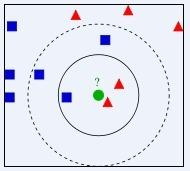如果K=3，绿色圆点的最近的3个邻居是2个红色小三角形和1个蓝色小正方形，少数从属于多数，基于统计的方法，判定绿色的这个待分类点属于红色的三角形一类。
如果K=5，绿色圆点的最近的5个邻居是2个红色三角形和3个蓝色的正方形，还是少数从属于多数，基于统计的方法，判定绿色的这个待分类点属于蓝色的正方形一类。
然后就是关于距离运算公式，如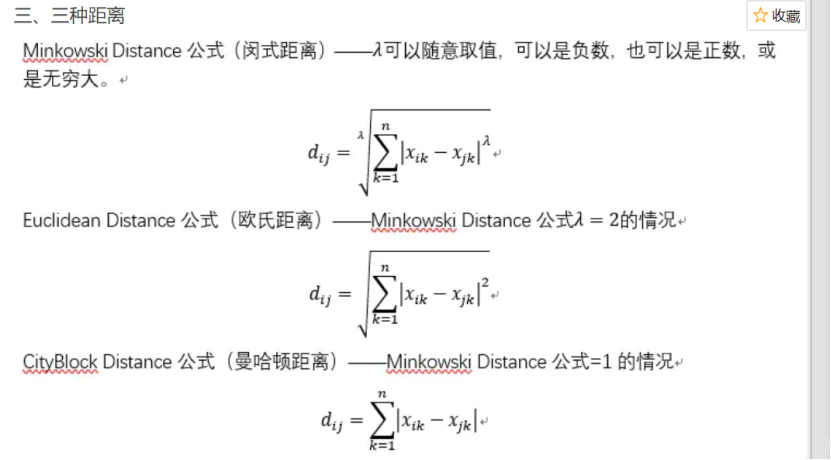然后就是步骤：
就是找个随便分为几部分给定k值，根据求出的距离选定距离最近的k个样本"""
“”"
import numpy as np
from matplotlib import pyplot as plt
#计算距离公式
def d_man(x, y):
d = np.sum(np.abs(x - y))#曼哈顿距离公式
return d
def d_euc(x, y):
d = np.sqrt(np.sum(np.square(x - y)))#欧式距离公式
return d
#sorted函数按照key值进行排序，key参数的值为一个函数，此函数只有一个参数且返回一个值用来进行比较
#reverse参数接受False 或者True 表示是否逆序；class_count.items()返回可遍历的(键, 值) 元组数组。
import operator
def majority_voting(class_count):
sorted_class_count = sorted(class_count.items(), key=operator.itemgetter(1), reverse=True)#itemgetter（a）获得第_a_个值,默认从小到大
return sorted_class_count
#生成示例数据
def create_data():
features = np.array(
[[2.88, 3.05], [3.1, 2.45], [3.05, 2.8], [2.9, 2.7], [2.75, 3.4],
[3.23, 2.9], [3.2, 3.75], [3.5, 2.9], [3.65, 3.6], [3.35, 3.3]]) #np.array构建二维数组且无需指针
labels = [‘A’, ‘A’, ‘A’, ‘A’, ‘A’, ‘B’, ‘B’, ‘B’, ‘B’, ‘B’]
return features, labels      #返回features,labels
def knn_classify(test_data, train_data, labels, k):
distances = np.array([])  # 创建一个空的数组存放距离
for each_data in train_data:  # 使用欧式距离计算数据相似度
d = d_euc(test_data, each_data)
distances = np.append(distances, d)      #append() 用于在列表末尾添加新的对象。
print(f"距离相似度distances={distances}")
sorted_distance_index = distances.argsort()  # 获取按距离大小排序后的索引(argsort提取索引)
sorted_distance = np.sort(distances)
print(f"查看sorted_distance_index{sorted_distance_index}")
print(f"查看sorted_distance{sorted_distance}")
r = (sorted_distance[k]+sorted_distance[k-1])/2  # 计算圆的半径

#选择距离最近的k个值
class_count = {}
for i in range(k):
vote_label = labels[sorted_distance_index[i]]  #根据索引找到距离对应的类别
class_count[vote_label] = class_count.get(vote_label, 0) + 1
#排序
final_label = majority_voting(class_count)
return final_label, r            #返回值

#生成训练样本
features, labels =create_data()
test_data = np.array([3.18, 3.15])
final_label, r = knn_classify(test_data, features, labels, 5)
print(final_label)
print®
#可视化
#极坐标方式表示圆
def circle(r, a, b):  # 为了画出圆，这里采用极坐标的方式对圆进行表示 ：x=rcosθ，y=rsinθ。
theta = np.arange(0, 2*np.pi, 0.01)
x = a+r * np.cos(theta)
y = b+r * np.sin(theta)
return x, y
k_circle_x, k_circle_y = circle(r, 3.18, 3.15)
plt.figure(figsize=(5, 5))
plt.xlim((2.4, 3.8))                  #x轴范围
plt.ylim((2.4, 3.8))                  #y轴范围
x_feature = list(map(lambda x: x, features))  # 返回每个数据的x特征值
y_feature = list(map(lambda y: y, features))
plt.scatter(x_feature[:5], y_feature[:5], c=“b”)  # 在画布上绘画出"A"类标签的数据点
plt.scatter(x_feature[5:], y_feature[5:], c=“g”)
plt.scatter([3.18], [3.15], c=“r”, marker=“x”)  # 待测试点的坐标为 [3.18，3.15]
plt.plot(k_circle_x, k_circle_y)



展开全文• 2.4 圆的生成—Bresenham算法 57 2.5 椭圆的生成 64 2.6 一般函数的光栅化 69 2.7 扫描转换—显示的生成 71 2.7.1 实时扫描转换 71 2.7.2 使用指针的简单活化边表 72 2.7.3 排序活化边表 72 2.7.4 使用链表...
• ## 谈谈站桩

千次阅读 2014-08-22 13:04:26
关于站桩总体要求，陈小旺老师把桩名叫做中定桩，这个桩名就明示了站桩要求：中定，为了配合太极拳套路架子规范，再把架子一些要求结合到站桩动作要求里面,比如含胸塌腰、沉肩坠肘、屈膝档、开档贵...
• 16.5.3 游戏帮助、关于、加载及结束界面设计与实现 402 16.6 游戏界面设计与实现 402 16.6.1 游戏界面框架设计 402 16.6.2 游戏界面实现 404 16.7 游戏界面中主要场景绘制及篮球运动 407 16.7.1 游戏...
• 　扇形面积S= lR，其中l是扇形的弧长，R是圆的半径． 三、典型例题剖析 例1、给出下面四个命题：①终边相同的角相等．②第一象限的角都是正角，③小于90°的角是锐角，④钝角是第二象限的角，其中正确命题的序号是__...
• 1.4　关于GNU规范语法扩展 10 1.5　用C语言构建一个可执行程序流程 11 1.6　本章小结 12 第2章 学习C语言预备知识 /14 2.1　计算机体系结构简介 14 2.1.1　贮存器 15 2.1.2　存储器 15 2.1.3　寄存器 16 2.1.4...
• - <code>mouseout</code> 触发判定也类似... 如果不是规整矩形，而是不规则多边形sprite 上面我们说情况都是基于规则矩形情况来说。如果不是一个规则矩形，而是一个不规则封闭多边形 或者...
• 由于数据元素在计算机存储空间中位置关系可能与逻辑关系不同，因此，为了表示存放在计算机存储空间中各数据元素之间逻辑关系（即前后件关系），在数据存储结构中，不仅要存放各数据元素信息，还需要存放各...算法
• ## java源码包---java 源码 大量 实例

千次下载 热门讨论 2013-04-18 23:15:26
关于数字签名：产生RSA密钥对(myKeyPair)，得到RSA密钥对，产生Signature对象,对用私钥对信息(info)签名，用指定算法产生签名对象，用私钥初始化签名对象，将待签名数据传送给签名对象(须在初始化之后)，用公钥...
• 有界变差函数的判定法 ------------571・连续的有界变差函数 ------------572.可求长曲线 --------§5.斯蒂尔切斯积分 ------------573.斯蒂尔切斯积分的定义 ------------574.斯蒂尔切斯积分存在的一般条 --------...
• 几何原本是一本关于几何里程碑式著作，它以十分严谨标准写成；每个命题 都是通过一个 用三段论链接形式论证 来合理化（虽然它们并不总是严格地遵守亚里士多德模式）。亚里士多德三段论逻辑 加上公理化方法...
• ## java源码包2

千次下载 热门讨论 2013-04-20 11:28:17
关于数字签名：产生RSA密钥对(myKeyPair)，得到RSA密钥对，产生Signature对象,对用私钥对信息(info)签名，用指定算法产生签名对象，用私钥初始化签名对象，将待签名数据传送给签名对象(须在初始化之后)，用公钥...
• ## java源码包3

千次下载 热门讨论 2013-04-20 11:30:13
关于数字签名：产生RSA密钥对(myKeyPair)，得到RSA密钥对，产生Signature对象,对用私钥对信息(info)签名，用指定算法产生签名对象，用私钥初始化签名对象，将待签名数据传送给签名对象(须在初始化之后)，用公钥...
• ## java源码包4

千次下载 热门讨论 2013-04-20 11:31:44
关于数字签名：产生RSA密钥对(myKeyPair)，得到RSA密钥对，产生Signature对象,对用私钥对信息(info)签名，用指定算法产生签名对象，用私钥初始化签名对象，将待签名数据传送给签名对象(须在初始化之后)，用公钥...
• 关于数字签名：产生RSA密钥对(myKeyPair)，得到RSA密钥对，产生Signature对象,对用私钥对信息(info)签名，用指定算法产生签名对象，用私钥初始化签名对象，将待签名数据传送给签名对象(须在初始化之后)，用公钥...